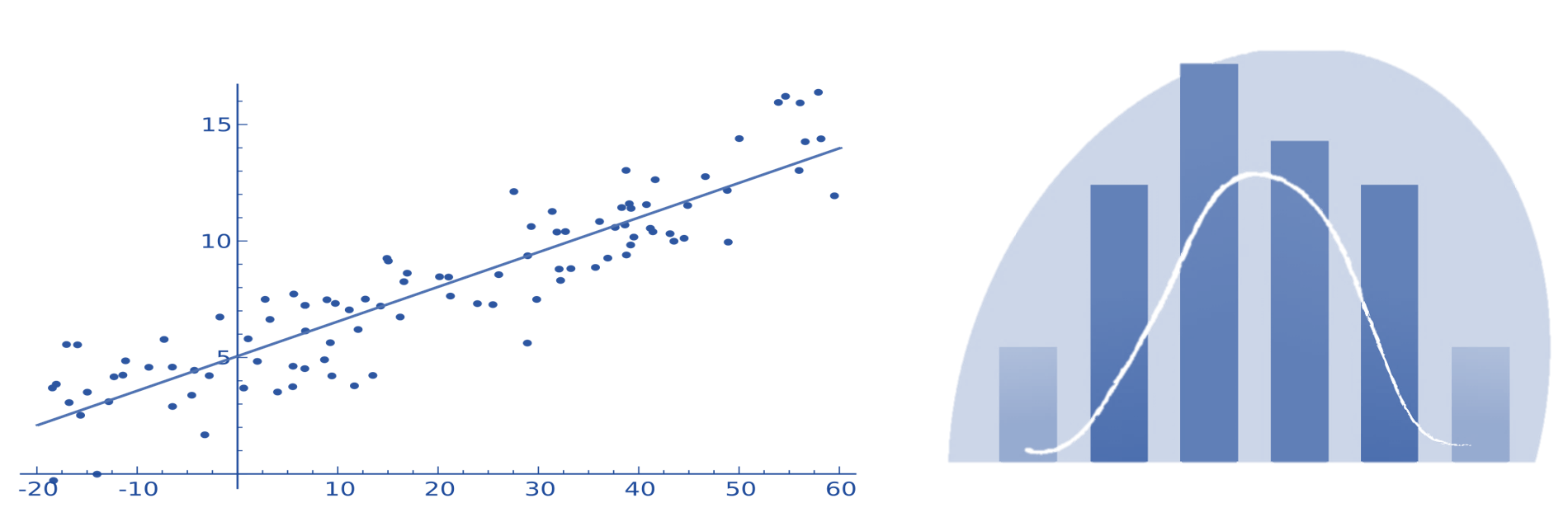# Mathematical statistics for biologists

General description

The aim of the course is to provide an in-depth knowledge of the basic methods of classical statistics and their application to biomedical data analysis. Statistical learning is a crucial tool for anyone who wants to make sense of their data and extract useful patterns from an array of information. This field of science combines both classical statistics and modern machine learning methods to create models that describe complex relationships between data, as well as to draw the necessary conclusions about the nature of this interaction.

Lectures
1. Basic definitions and principles of classical statistics methods.

Statistical learning, basic definitions. Null and alternative hypotheses. Statistical significance, effect size.

2. The main tools of the researcher in conducting statistical analysis.

Overview of the main tools for performing statistical training. Programming languages R and Python as the most flexible and powerful options.

3. Methods of comparing means between 2 groups.

Parametric and nonparametric methods for comparing means between 2 groups. Welch's method. Student's method. Checking normality and homoscedasticity of data.

4. Method of one-factor analysis of variance.

Basic principles of the method. Features of application. Checking the basic assumptions of the method. Correction for multiple comparisons.

5. Two-factor analysis of variance. Analysis of variance with mixed effects.

Features of the application of two-factor analysis of variance. Multivariate analysis of variance. Analysis of variance with repeated measurements.

6. Assessment of the strength of the relationship between variables.

Correlation and covariance. Correlation as the magnitude of the effect. The main types of correlations. Partial correlation.

7. Estimation of the frequency response between variables.

Features of the chi-square method. Calculation of the expected frequency of the phenomenon. Fisher's exact method.

8. Linear regression method.

Basic principles of linear regression methods. Method of least squares. Gradient descent method for determining coefficients.

9. The main types of regularization.

Definition of regularization. Lasso regularization. Ridge regularization. Elastic grid.

10. Method of logistic regression.

Sigmoid function. Linear combination of predictors. Odds ratio and interpretation of regression coefficients.

Seminars
1. Python ecosystem for data analysis.
2. Comparison of averages between groups.
3. Correlation analysis.
4. Analysis of variance.
5.Linear and logistic regression.Level
Bachelor and master students
Lectures
10 lectures
Practical classes
5 classes
Duration
1 month
Language
Ukrainian
Certificate
1 credit ECTS
Lecturers

## Dmytro Stroy

Researcher in the Institute of Physiology of A. Bogomoletz, Kyiv

Предмети курсу